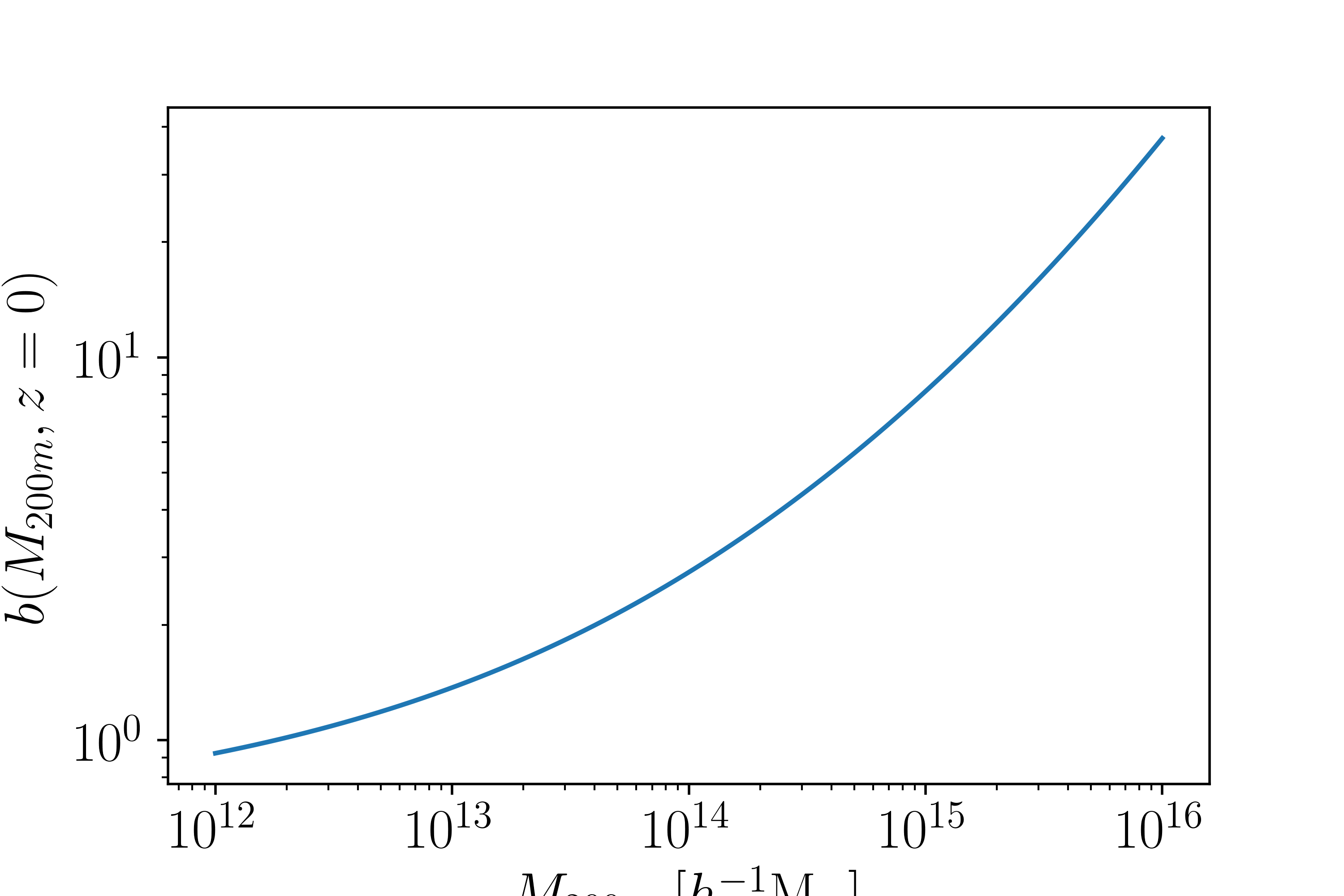# Halo Bias¶

Halos, which host galaxies and galaxy clusters, are biased tracers of the matter density field. This means at large scales the correlation function is

$\xi_{\rm hm}(R) = b\xi_{\rm mm}$

where the bias is a function of mass (and cosmological parameters). This module implements the Tinker et al. 2010 halo bias model, which is accurate to 6%. Other biases will be available in the future. To use this you would do:

from cluster_toolkit import bias
mass = 1e14 #Msun/h
Omega_m = 0.3
#Assume that k and P_linear came from somewhere, e.g. CAMB or CLASS
bias = bias.bias_at_M(mass, k, P_linear, Omega_m)


Note

The bias can use $$\Delta\neq 200$$ as an argument delta=200.

This module also allows for conversions between mass and RMS density variance $$\sigma^2$$ and peak height $$\nu$$.

from cluster_toolkit import bias
mass = 1e14 #Msun/h
Omega_m = 0.3
#Assume that k and P_linear came from somewhere, e.g. CAMB or CLASS
sigma2= bias.sigma2_at_M(mass, k, P_linear, Omega_m)
nu = bias.nu_at_M(Mass, k, P_linear, Omega_m)


The bias as a function of mass is seen here for a basic cosmology: